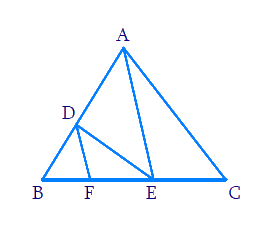# Ex.6.2 Q4 Triangles Solution - NCERT Maths Class 10

Go back to  'Ex.6.2'

## Question

In Figure, $$DE || AC$$ and $$DF || AE.$$ Prove that

\begin{align}\frac{BF}{FE}=\frac{BE}{EC}\end{align}Video Solution
Triangles
Ex 6.2 | Question 4

## Text Solution

Reasoning:

As we know if a line is drawn parallel to one side of a triangle to intersect the other two sides in distinct points, the other two sides are divided in the same ratio.

Steps:

In $$\Delta ABC$$

\begin{align} & DE||AC \end{align}

\begin{align}\frac{BD}{AD}=\frac{BE}{EC}\;\dots\text{(i)} \\ \end{align}

In\begin{align}\Delta \,ABE \end{align}

\begin{align}&DF||AE \\ &\frac{BD}{AD}=\frac{BF}{FE}\;\dots\text{(ii)} \end{align}

From $$\rm (i)$$ and $$\rm (ii)$$

\begin{align} & \frac{BD}{AD}=\frac{BE}{EC}=\frac{BF}{FE} \\ & \frac{BE}{EC}=\frac{BF}{FE} \\ \end{align}

Learn from the best math teachers and top your exams

• Live one on one classroom and doubt clearing
• Practice worksheets in and after class for conceptual clarity
• Personalized curriculum to keep up with school